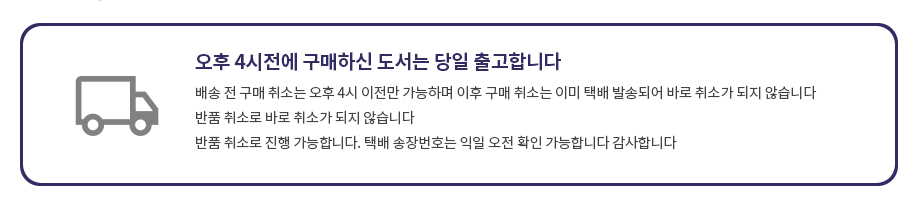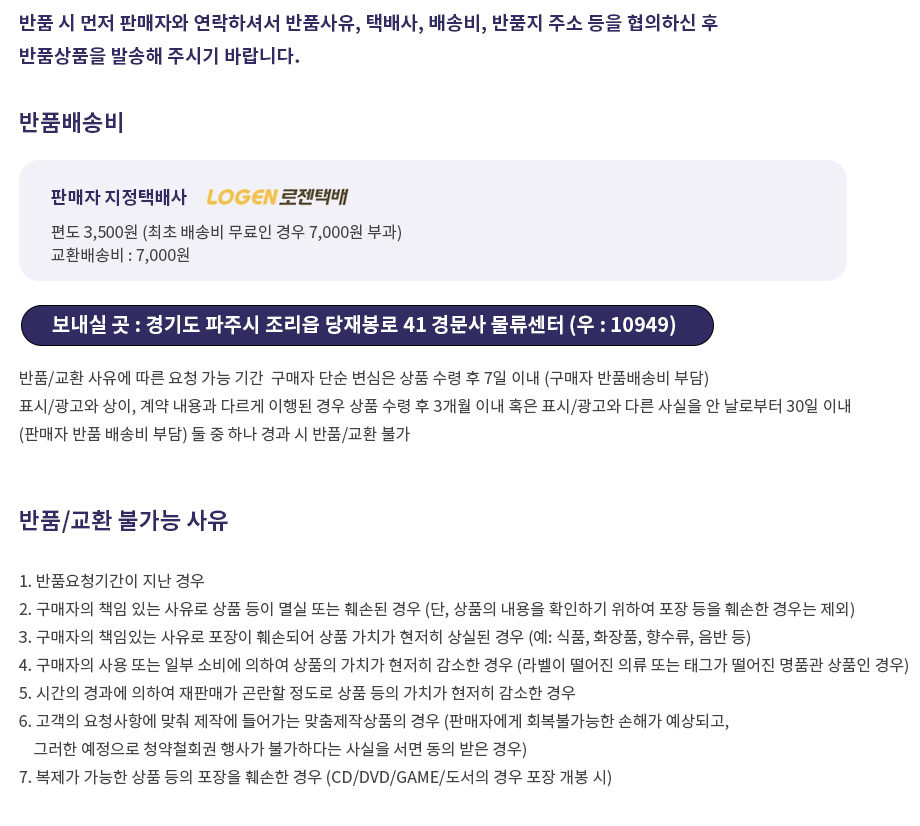## 로그인이필요합니다## 도서를 검색해 주세요.

원하시는 결과가 없으시면 문의 주시거나 다른 검색어를 입력해보세요.

•상품상세

## Measure and Integral: An Introduction to Real Analysis 요약정보 및 구매

상품 선택옵션 0 개, 추가옵션 0 개

사용후기 0 개
지은이 Richard L. Wheeden 1977-05-31 1판 288 9780824764999 구매가능 42,000원 0점 주문시 결제

### 선택된 옵션

• Measure and Integral: An Introduction to Real Analysis
+0원

## 관련상품

• This volume develops the classical theory of the Lebesgue integral and some of its applications. The integral is initially presented in the context of n-dimensional Euclidean space, following a thorough study of the concepts of outer measure and measure. A more general treatment of the integral, based on an axiomatic approach, is later given. Closely related topics in real variables, such as functions of bounded variation, the Riemann-Stieltjes integral, Fubini's theorem, L(p)) classes, and various results about differentiation are examined in detail. Several applications of the theory to a specific branch of analysis--harmonic analysis--are also provided. Among these applications are basic facts about convolution operators and Fourier series, including results for the conjugate function and the Hardy-Littlewood maximal function. Measure and Integral: An Introduction to Real Analysis provides an introduction to real analysis for student interested in mathematics, statistics, or probability. Requiring only a basic familiarity with advanced calculus, this volume is an excellent textbook for advanced undergraduate or first-year graduate student in these areas. ﻿
• Preliminaries Points and Sets in Rn Rn as a Metric Space Open and Closed Sets in Rn: Special Sets Compact Sets; The Heine-Borel Theorem Functions Continuous Functions and Transformations The Riemann Integral Exercises Function of Bounded Variation; The Riemann-Stieltjes Integral Functions of Bounded Variation Rectifiable Curves The Reiman-Stieltjes Integral Further Results About the Reimann-Stieltjes Integrals Exercises Lebesgue Measure and Outer Measure Lebesgue Outer Measures; The Cantor Set. Lebesgue Measurable Sets Two Properties of Lebesgue Measure Characterizations of Measurability Lipschitz Transformations of Rn A Nonmeasurable Set. Exercises Lebesgue Measurable Functions Elementary Properties of Measurable Functions. Semicontinuous Functions Properties of Measurable Functions; Egorov's Theorem and Lusin's Theorem Convergence in Measure Exercises The Lebesgue Integral Definition of the Integral of a Nonnegative Function Properties of the Integral The Integral of an Arbitrary Measurable f A Relation Between Riemann-Stieltjes and Lebesgue Integrals; the LP Spaces, 0
• 학습자료

등록된 학습자료가 없습니다.

정오표

등록된 정오표가 없습니다.

사용후기가 없습니다.

상품문의가 없습니다.

• ## 배송/교환정보

### 배송정보### 교환/반품 정보### 선택된 옵션

• Measure and Integral: An Introduction to Real Analysis
+0원